Bulbs and batteries

Series and parallel

The brightness of a bulb shows how much power it is using.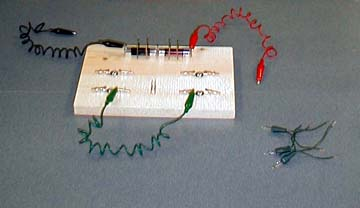A pine board with a battery holder made of nails and alligator clips is used to hold Christmas tree lights in series or parallel.

Material

• Christmas tree bulbs from series chains of 50 bulbs. For the best prices, buy these after Christmas.
• 2 x 1.5 volt AA batteries
• aluminum foil
• A breadboard to connect the bulbs and batteries
• or a battery holder and alligator clips.

Assembly

Cut the lights apart leaving 10 cm (3") of wire connected to each bulb.
Cut the plastic insulation off the last 1 cm of wire, 0.5 inch.
Build a breadboard by screwing alligator clips onto a wooden plank see above.
Add a battery holder by driving nails into the plank.

To Do and Notice

Symbols

We'll use the symbol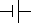for a battery, where the large plate indicates the positive end,

and the symbol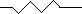for a bulb or resistor.

Connect one Christmas tree bulb in series with two batteries.
Notice that it glows brightly.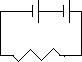One bulb in series with two batteries.

Stick an aluminum foil pad between the batteries, have part of the foil stick up so that it can be grabbed by an alligator clip.
Connect the bulb to one battery, notice that the bulb glows much brighter with two batteries than with one.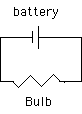Connect two bulbs in series with two batteries.
Notice that the bulbs are about as bright as one bulb in series with one battery.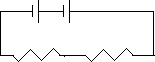Two bulbs in series with two batteries.

Connect two bulbs in parallel with two batteries.
Notice that the bulbs are about as bright as one bulb in series with two batteries.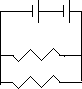Two bulbs in parallel.

What's Going On?

One battery produces a voltage difference of 1.5 volts.

The voltage across two batteries in series is the sum of the voltages of each battery.(Connect the negative terminal of one battery to the positive terminal of the other.)

The bulb is a resistor.
When a voltage is applied across a resistor a current flows through the resistor. I= V/R
The larger the voltage the higher the current. The current is linearly proportional to the voltage.

When a current flows through a voltage drop power is dissipated.
The power dissipated by a lightbulb produces light and heat.
The power is the product of the voltage and the current. P = V*I(See the Math Root.)

Thus two batteries produce twice the voltage, 3 volts, and twice the current through one bulb than one battery.
The power of the bulb is thus four times greater with two batteries as with one.

When two bulbs are connected in series with two batteries, each bulb shares the voltage drop equally. So each bulb has half the total voltage across it. Each bulb has the voltage of one battery across it. And each bulb glows the same as if it were connected to one battery. The bulbs are equally bright and dim. The current through every point in a single series loop is the same. So the same current flows through both bulbs.

When two bulbs are connected in parallel to two batteries then each bulb has the full voltage across it. Each bulb glows as brightly as if it were connected to two batteries. The current flowing through the batteries splits, since the bulbs are identical, half the current flows through each bulb.

Math Root

Ohm's law relates the current flowing through a bulb, I, to the voltage across the bulb, V, and the resistance of the bulb,R.

I = V/R

Before you apply ohms law "point to the resistor" that you are applying the law to.

The current direction is the direction of flow that positive charges would have: that is, from the higher voltage end of the bulb to the lower voltage end.

The law for the power dissipated by the bulb in watts is

P = VI

Where:

V is the voltage across the bulb in volts and
I is the current through it in amps.

Substituting ohm's law into the power law we find:

P = V2/R

So when we double the voltage by using two batteries the power goes like the voltage squared and quadruples.

Note that P for power is the Product of voltage and current.

Note that R for resistance R = V/I is the ratio of voltage to current.

 Scientific Explorations with Paul Doherty © 2000 7 October 2000